# Colleges with the highest SAT scores in Oregon

Top 10 colleges in Oregon with the highest SAT scores
Looking for the colleges with the highest SAT scores in Oregon? Well you're in luck! We've compiled a national college database and have created a list of the top 10 universities with the highest SAT scores in Oregon. These are the schools whose applicants had the highest average SAT scores in Oregon. And, since these tests are meant to determine academic prowess, they are arguably the schools with the most academically proficient students. You could even say these are the best colleges in Oregon. We also include each college's ACT scores and acceptance rate so that you can see where you would have the easiest or hardest time getting in. Read on to find out more.

## Reed College SAT scores

The average SAT score for Reed College is 1420.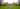The average SAT score of 1420 breaks down into:

• SAT math: 710

The average ACT score for Reed College is 32 and their acceptance rate is 35%.

## Lewis and Clark College SAT scores

The average SAT score for Lewis and Clark College is 1303.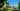The average SAT score of 1303 breaks down into:

• SAT math: 640

The average ACT score for Lewis and Clark College is 28 and their acceptance rate is 73.8%.

## University of Portland SAT scores

The average SAT score for University of Portland is 1260.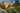The average SAT score of 1260 breaks down into:

• SAT math: 620

The average ACT score for University of Portland is 27 and their acceptance rate is 61.9%.

## Willamette University SAT scores

The average SAT score for Willamette University is 1230.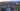The average SAT score of 1230 breaks down into:

• SAT math: 620

The average ACT score for Willamette University is 28 and their acceptance rate is 83.6%.

## Oregon State University SAT scores

The average SAT score for Oregon State University is 1195.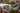The average SAT score of 1195 breaks down into:

• SAT math: 600

The average ACT score for Oregon State University is 25 and their acceptance rate is 80.6%.

## University of Oregon SAT scores

The average SAT score for University of Oregon is 1185.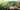The average SAT score of 1185 breaks down into:

• SAT math: 585

The average ACT score for University of Oregon is 25 and their acceptance rate is 83.4%.

## George Fox University SAT scores

The average SAT score for George Fox University is 1150.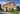The average SAT score of 1150 breaks down into:

• SAT math: 560

The average ACT score for George Fox University is 23 and their acceptance rate is 78.3%.

## Pacific University Oregon SAT scores

The average SAT score for Pacific University Oregon is 1150.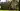The average SAT score of 1150 breaks down into:

• SAT math: 570

The average ACT score for Pacific University Oregon is 24 and their acceptance rate is 79%.

## Portland State University SAT scores

The average SAT score for Portland State University is 1119.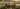The average SAT score of 1119 breaks down into:

• SAT math: 550

The average ACT score for Portland State University is 22 and their acceptance rate is 90%.

## Linfield College SAT scores

The average SAT score for Linfield College is 1110.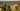The average SAT score of 1110 breaks down into:

• SAT math: 550